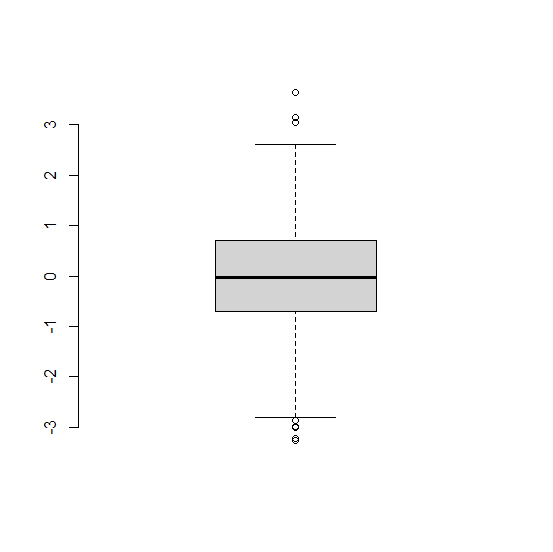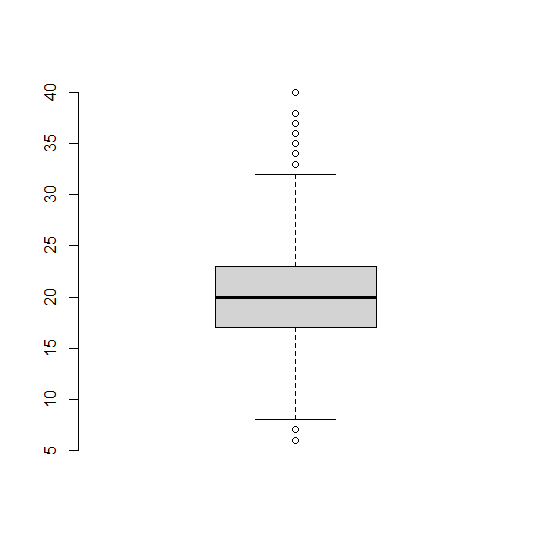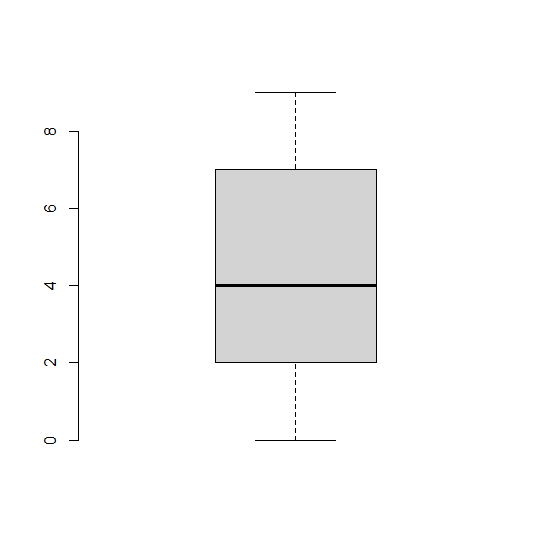# How to create a boxplot in base R without any axes except Y?

R ProgrammingServer Side ProgrammingProgramming

The boxplot function in base R helps us to create the boxplot without any hustle but this plot is covered with a square bracket and also takes the Y-axis labels on left-hand side. We can get rid of this square bracket without making an impact on the Y-axis labels. For this purpose, we need to use frame.plot = FALSE argument inside the boxplot function.

## Example1

> x<-rnorm(1000)
> boxplot(x,frame.plot=FALSE)

## Output:## Example2

> y<-rpois(5000,20)
> boxplot(y,frame.plot=FALSE)

## Output:## Example3

> z<-sample(0:9,500,replace=TRUE)
> boxplot(z,frame.plot=FALSE)

## Output: